# Thermochemistry – online MCQ test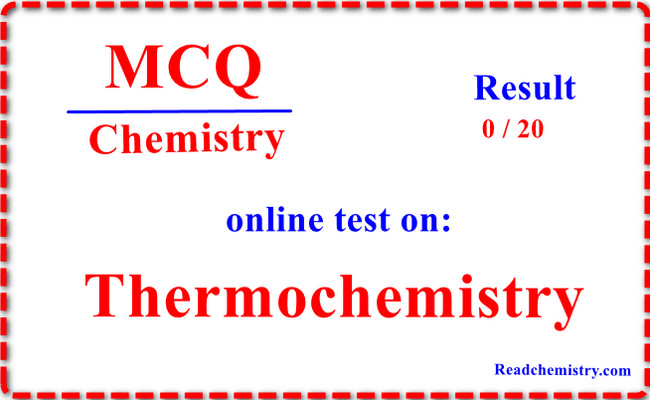## Online MCQ test on Thermochemistry

– In this topic we offer you, online MCQ test in the fundmental of Thermochemistry.

– It is a simple test to measure the strength of your knowledge in the basics of understanding the topics of Thermochemistry

– The test consists of only (15) questions (multiple choice) you must answer all of them so that your final score appears automatically at the top of the topic.

– you will pass the Exam If get 70% or more

– If you fail the test, you can retake the test again by reloading the page

– Let’s start the test, good luck.

## Starting the test of Thermochemistry

``` ```

``` Results - (adsbygoogle = window.adsbygoogle || []).push({}); Congratulation(adsbygoogle = window.adsbygoogle || []).push({}); Try again#1. For an endothermic reaction _____ ΔH is –ve ΔH is –ve ΔH is +ve ΔH is +ve ΔE is –ve ΔE is –ve ΔH is zero ΔH is zero #2. The bond energy depends upon _____ size of the atom size of the atom electronegativity electronegativity bond length bond length all of these all of these #3. The heat of neutralisation of a strong acid and a strong base is always _____ zero zero constant constant positive positive changing changing #4. The apparatus used to measure heat changes during chemical reactions is called _____ polarimeter polarimeter colorimeter colorimeter calorimeter calorimeter none of these none of these #5. The Kirchoff’s equation is ________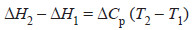(a) (a)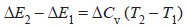(b) (b) both (a) , (b) both (a) , (b) neither (a) nor (b) neither (a) nor (b) #6. Hess’s law is used to determine _________ heat of formation of substances which are otherwise difficult to measure heat of formation of substances which are otherwise difficult to measure heat of transition heat of transition heats of various other reactions like dimerization heats of various other reactions like dimerization all of the above all of the above #7. In an exothermic reaction, the internal energy of the products is _______ internal energy of the reactants greater than greater than lesser than lesser than equal to equal to none of these none of these #8. For exothermic reactions, ΔH is _______ while for endothermic reactions it is _______. positive, negative positive, negative positive, positive positive, positive negative, negative negative, negative negative, positive negative, positive #9. The change in enthalpy when one mole of a substance is dissolved in a specified quantity of solvent at a given temperature is called heat of reaction heat of reaction heat of combustion heat of combustion heat of solvation heat of solvation heat of solution heat of solution #10. The branch of chemistry which deals with the heat changes caused by chemical reactions is called thermodynamics thermodynamics thermal chemistry thermal chemistry thermochemistry thermochemistry none of these none of these #11. ΔH° represent the enthalpy change _________ at 0°C and 1 atm pressure at 0°C and 1 atm pressure at 0 K and 1 atm pressure at 0 K and 1 atm pressure at 25 K and 1 atm pressure at 25 K and 1 atm pressure none of these none of these #12. The thermochemical equations may be ________ multiplied multiplied added added subtracted subtracted all of these all of these #13. The enthalpy of a system is defined by the relation..... H = E + P V H = E + P V H = E – P V H = E – P V E = H + P V E = H + P V P V + E – H P V + E – H #14. The calorific value is defined as the amount of heat produced in calories when _______ one gram one gram one mole one mole 100 grams 100 grams 1 kg 1 kg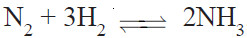#15. For the reaction above , the change in enthalpy is given by ____ ΔH = ΔE – 2 R T ΔH = ΔE – 2 R T ΔH = ΔE + 2 R T ΔH = ΔE + 2 R T ΔH = ΔE + 3 R T ΔH = ΔE + 3 R T ΔH = ΔE + R T ΔH = ΔE + R T #16. Which one of the following is correct for a reaction aA + bB → cC + dD where Δn = (c + d) – (a + b) PΔV = ΔH – ΔE PΔV = ΔH – ΔE ΔH = ΔE + Δn R T ΔH = ΔE + Δn R T Δn R T = ΔH – ΔE Δn R T = ΔH – ΔE all of these all of these #17. Which of the following always has a negative value? heat of formation heat of formation heat of reaction heat of reaction heat of combustion heat of combustion heat of solution heat of solution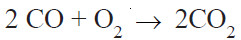#18. The enthalpy change in the reaction above is termed as _____ enthalpy of reaction enthalpy of reaction enthalpy of fusion enthalpy of fusion enthalpy of formation enthalpy of formation enthalpy of combustion enthalpy of combustion #19. In an exothermic reaction the heat energy is _______ while in endothermic reaction it is _______. released, released released, released released, absorbed released, absorbed absorbed, released absorbed, released absorbed, absorbed absorbed, absorbed #20. The fuel efficiency of methane (mol mass = 16) is _______ that of ethane (mol mass = 30). lesser than lesser than greater than greater than equal to equal to none of these none of these Finish ```
``` ```

Click on the word (Finish) to see the test result

## MCQs test onThermochemistry

– Our multiple choice questions and answers (MCQs) focus on all areas of Chemistry covering all chemistry topics.

– One can read MCQs on Chemistry here. These topics are chosen from a collection of the most authoritative and best reference books on Chemistry.

– One should spend 1 hour daily practicing these MCQs for 2-3 months to learn and assimilate Chemistry subject comprehensively.

– This way of systematic learning will prepare anyone easily for Chemistry – Class exams, contests, online tests, quizzes, MCQ-tests, viva-voce, interviews, and certifications.

– These MCQs are organized chapterwise and each Chapter is futher organized topicwise.

– Every MCQ set focuses on a specific topic of Chapter in Chemistry.

## Who should Practice MCQ Chemistry ?

(1) Students who are preparing for various school tests such as unit-tests, term-tests, semester tests, half-yearly and yearly tests and examinations on Chemistry

(2) Students who are preparing for Online/Offline Tests/Contests in Chemistry.

(3) Students who wish to sharpen their knowledge of Chemistry.

(4) Students who are studying in CBSE, ICSE, IGCSE schools as well as various State Board schools.

(5) Students who are preparing for competitive examinations such as NTSE, Olympiad.

(6) Students who are preparing for Central board examinations in Chemistry namely CBSE, ICSE, and NIOS Board.

(7)Students who are preparing for State board examinations in Chemistry such as PUC, Intermediate exams, etc.

(8) Students who are preparing for engineering and medical entrance examinations like NEET, IIT JEE, AIIMS, JIPMER & SAT Exams in Chemistry.